# NIUHE

## Machine translation with attention

• We are compressing a lot of information in a finite-sized vector.
• Gradients have a long way to travel. Even LSTMs forget!

Solution

• Represent a source sentence as a matrix
• Solve the capacity problem
• Generate a target sentence from a matrix
• Solve the gradient flow problem

Sentences as Matrices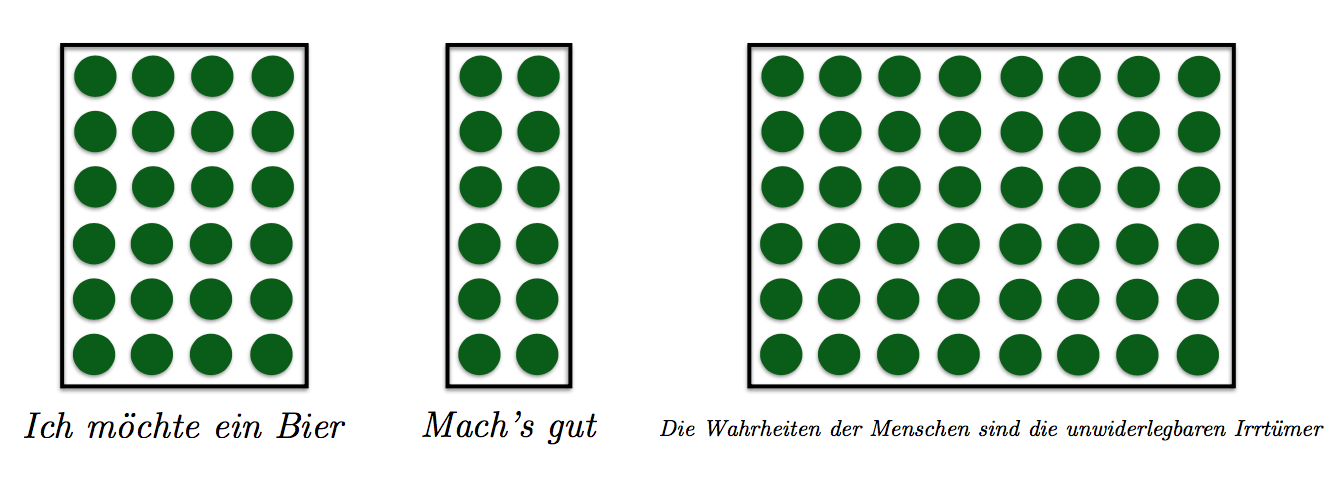Question: How do we build these matrices?

### With Concatenation

• Each word type is represented by an n-dimensional vector
• Take all of the vectors for the sentence and concatenate them into a matrix
• Simplest possible model
• So simple that no one publish how well/badly it works!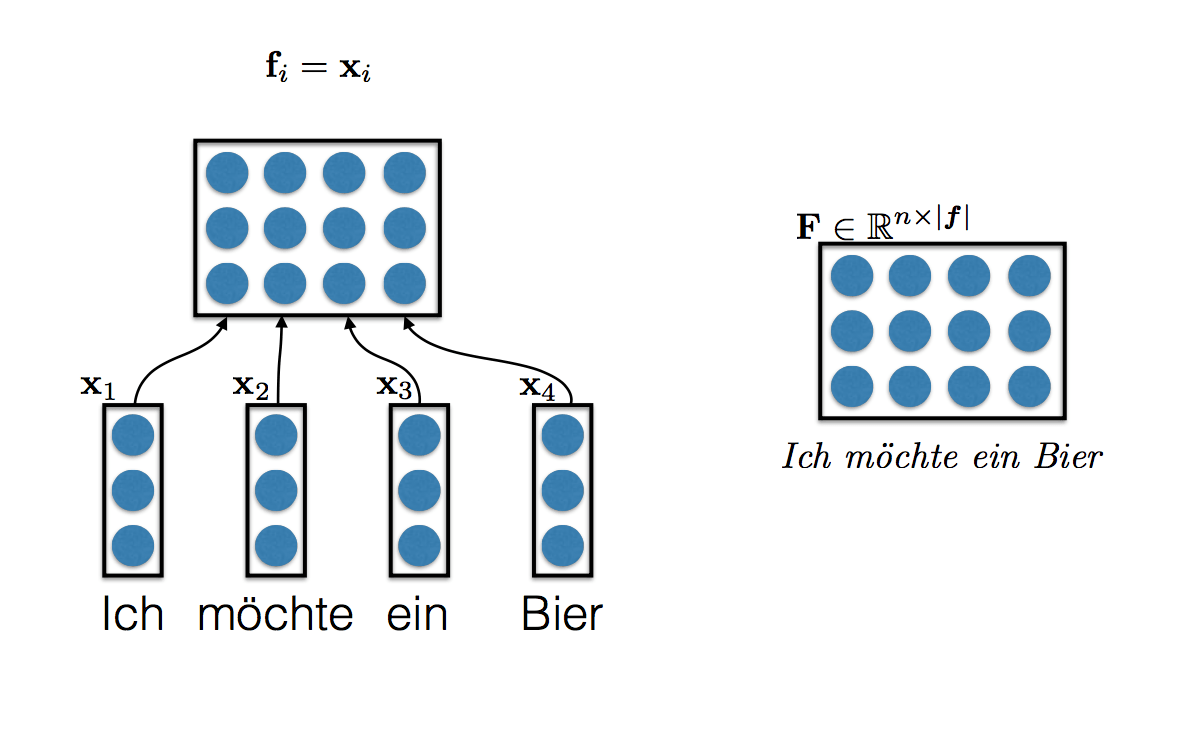### With Convolutional Nets

• Apply convolutional networks to transform the naive concatenated matrix to obtain a context-dependent matrix
• Note: convnets usually have a "pooling" operation at the top level that results in a fixed-sized representation. For sentences, leave this out.
• Papers
• Gehring et al., ICLR 2016
• Kalchbrenner and Blunsom, 2013### With Bidirectional RNNS

• By far the most widely used matrix representation, due to Bahdanau et al (2015)
• One column per word
• Each column (word) has two halves concatenated together:
• a “forward representation”, i.e., a word and its left context
• a “reverse representation”, i.e., a word and its right context
• Implementation: bidirectional RNNs (GRUs or LSTMs) to read f from left to right and right to left, concatenate representations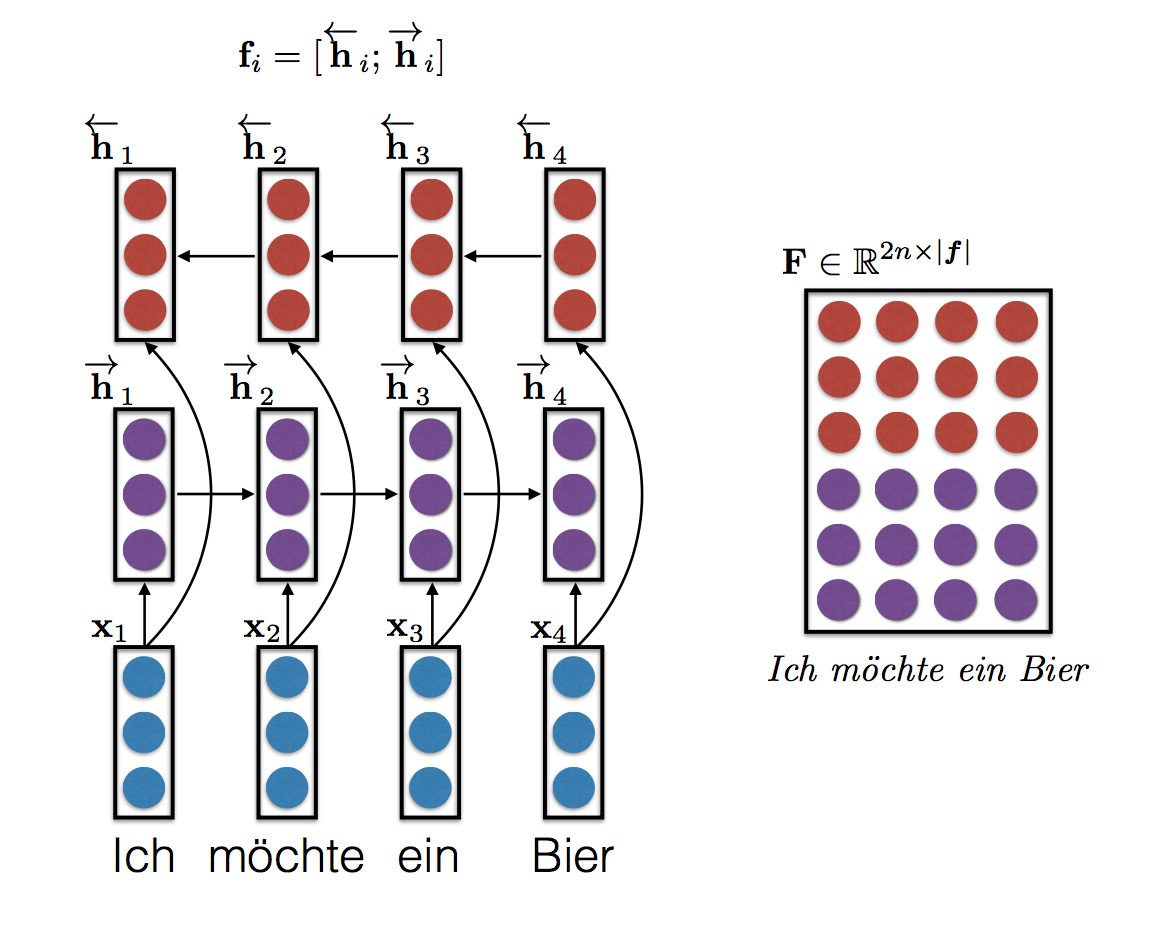Where are we in 2017?

There are lots of ways to construct $F$

• Very little systematic work comparing them

• There are many more undiscovered things out there

• convolutions are particularly interesting and under-explored
• syntactic information can help (Sennrich & Haddow, 2016; Nadejde et al., 2017), but many more integration stregration strategies are possible
• try something with phrase types instead of word types?

Multi-word expressions are a pain in the neck .

### Attention

Bahdanau et al. (2015) were the ﬁrst to propose using attention for translating from matrix-encoded sentences.

High-Level Idea

• Generate the output sentence word by word using an RNN
• At each output position $t$, the RNN receives two inputs (in addition to any recurrent inputs)
• a ﬁxed-size vector embedding of the previously generated output symbol $e_{t-1}$
• a ﬁxed-size vector encoding a “view” of the input matrix
• How do we get a ﬁxed-size vector from a matrix that changes over time?
• Bahdanau et al: do a weighted sum of the columns of $F$ (i.e., words) based on how important they are at the current time step. (i.e., just a matrix-vector product $Fa_t$ )
• The weighting of the input columns at each time-step ($a_t$) is called attention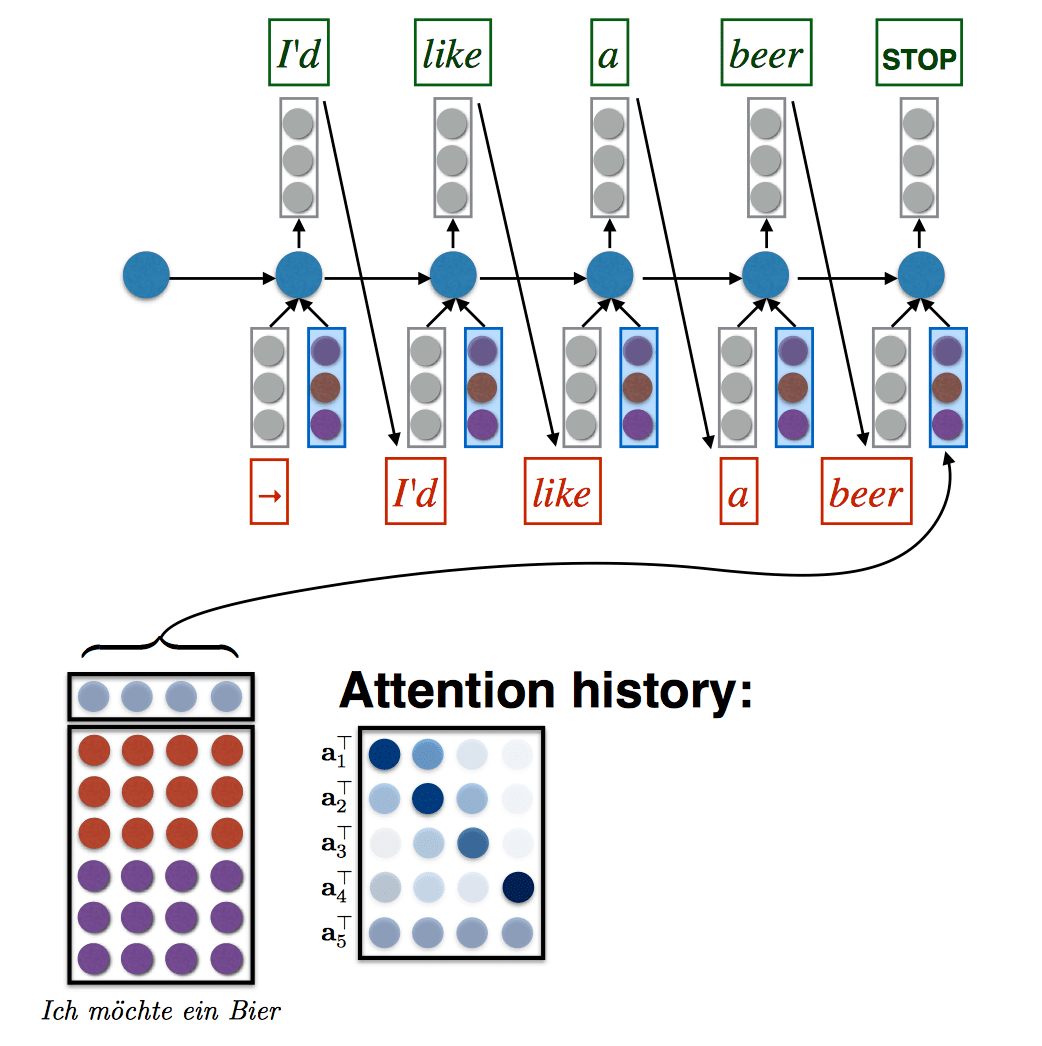Compute Attention

At each time step (one time step = one output word), we want to be able to “attend” to different words in the source sentence

• We need a weight for every column: this is an |$f$|-length vector a $a_t$
• Here is Bahdanau et al.’s solution
• Use an RNN to predict model output, call the hidden states $s_t$
• At time $t$ compute the expected input embedding $r_t = Vs_{t-1}$
• Take the dot product with every column in the source matrix to compute the nonlinear attention energy. $e_t = v^T\tanh(WF+r_t)$
• Exponentiate and normalize to 1: $a_t = softmax(u_t)$
• Finally, the input source vector for time t is $c_t = Fa_t$

The overall algorithm: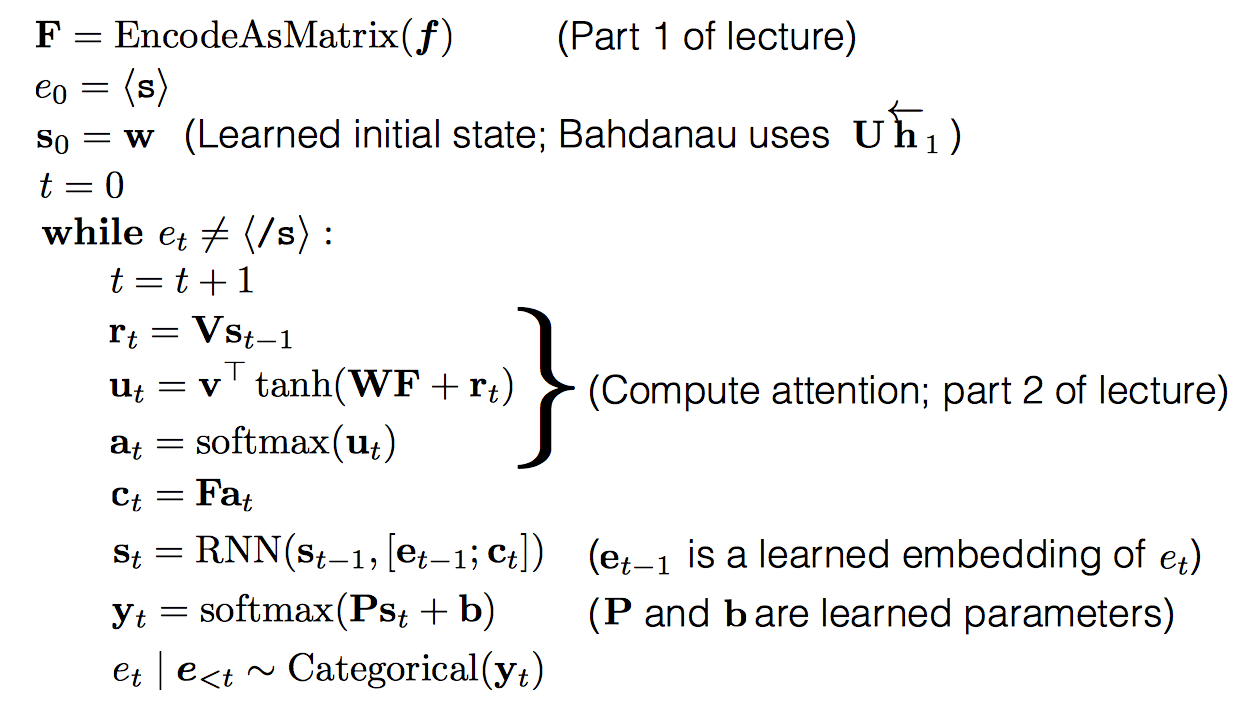Add attention to seq2seq translation: +11 BLEU

Model Variant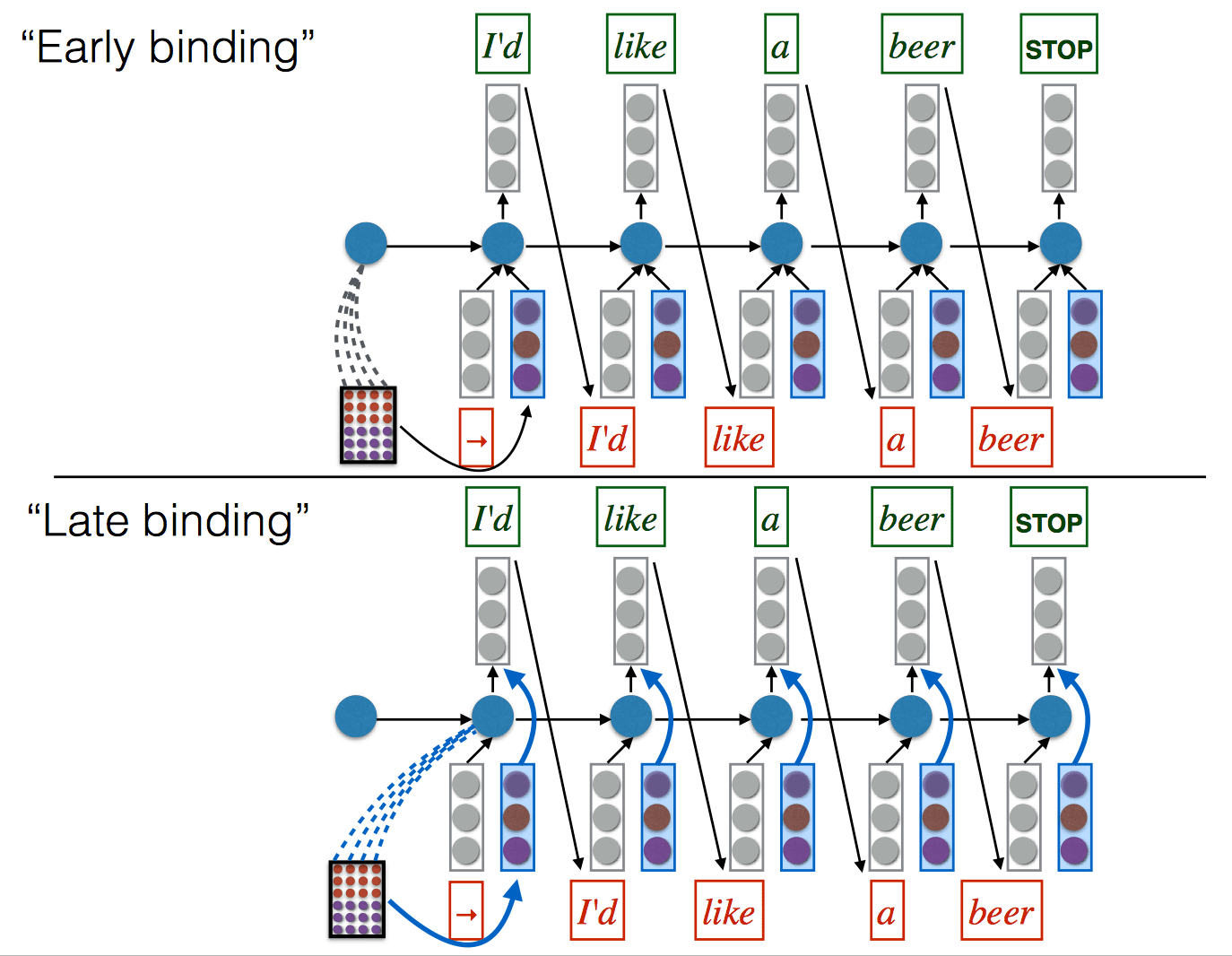Summary

• Attention is closely related to “pooling” operations in convnets (and other architectures)
• Bahdanau’s attention model seems to only cares about “content”
• No obvious bias in favor of diagonals, short jumps, fertility, etc.
• Some work has begun to add other “structural” biases (Luong et al., 2015; Cohn et al., 2016), but there are lots more opportunities
• Attention weights provide interpretation you can look at

## Image caption generation with attention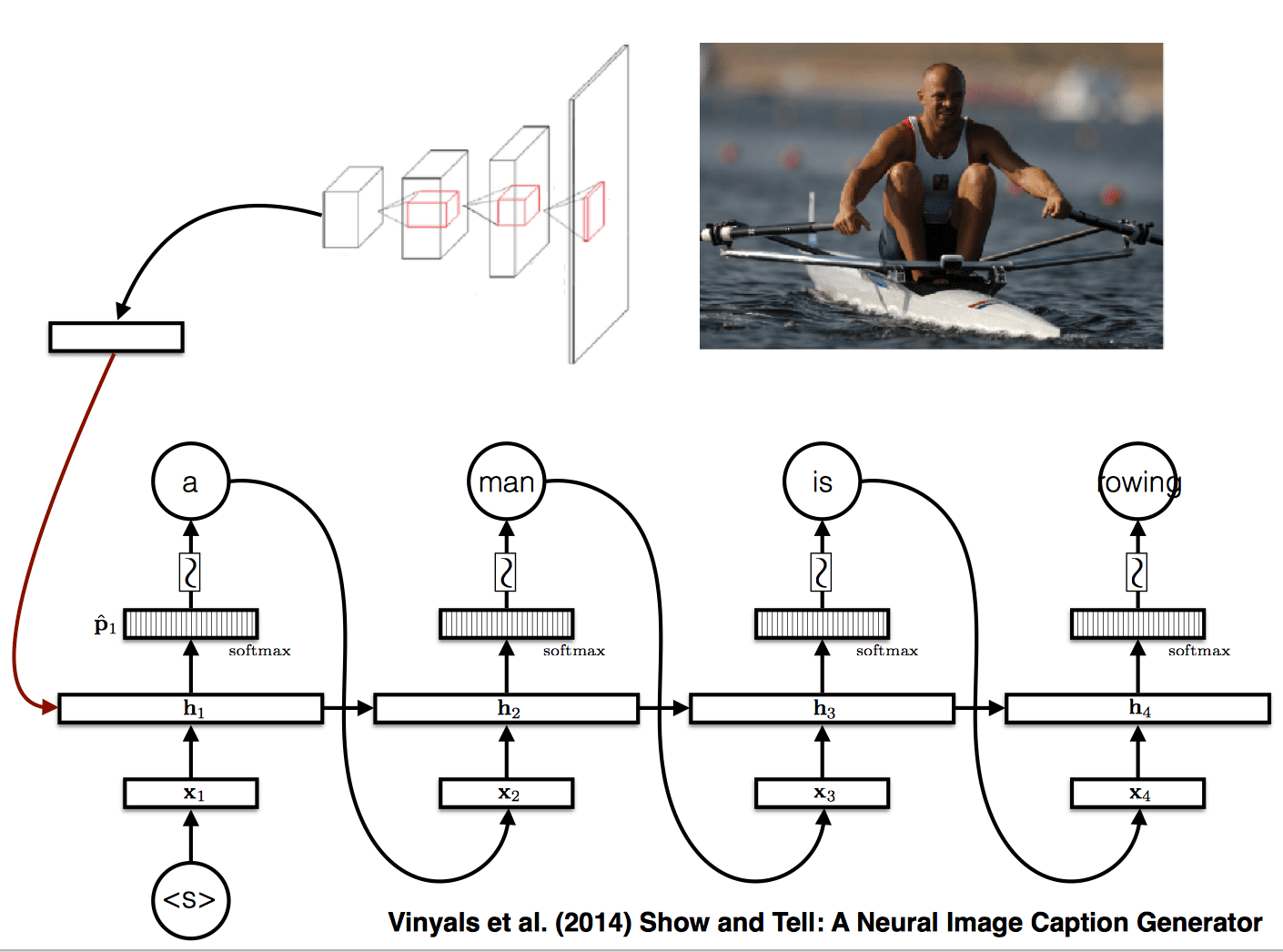Regions in ConvNets

Each point in a “higher” level of a convnet deﬁnes spatially localised feature vectors(/matrices).

Xu et al. calls these “annotation vectors”, $a_i$ , $i\in \{1, . . . , L\}$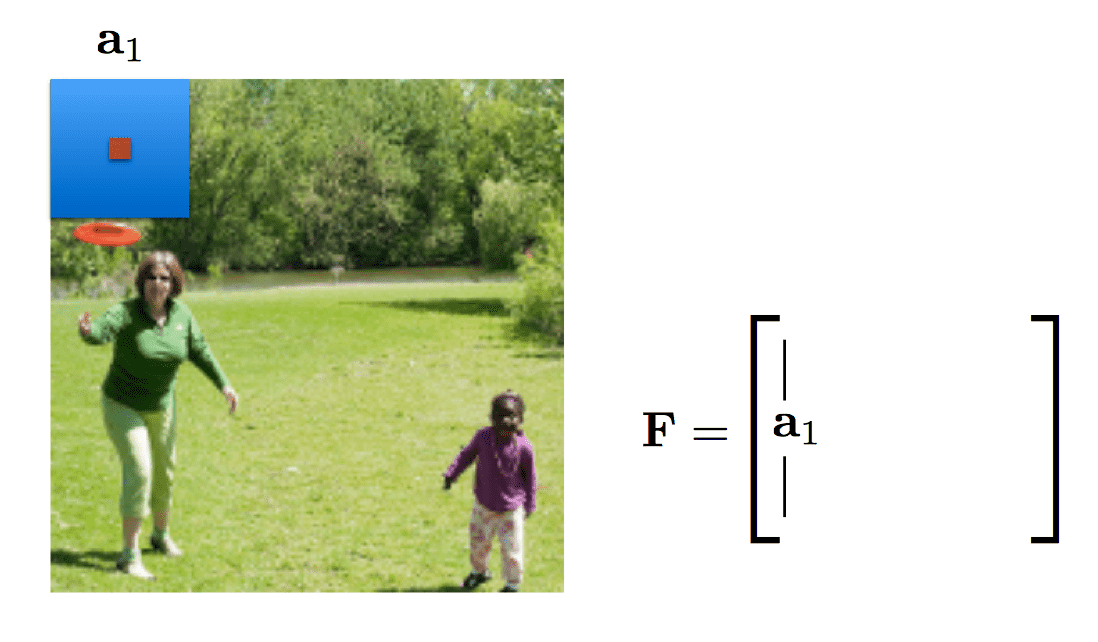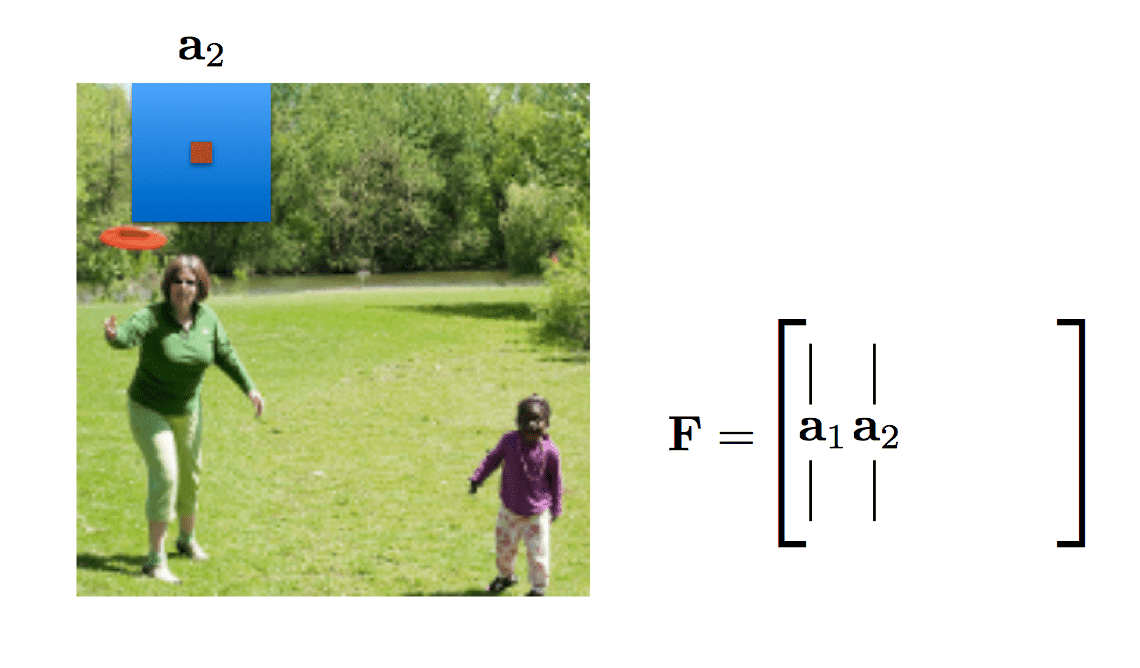Attention “weights” ( $a_t$ ) are computed using exactly the same technique as discussed above.

• Deterministic soft attention (Bahdanau et al., 2014)

$c_t = Fa_t$

• Stochastic hard attention (Xu et al., 2015)

$s_t \sim Categorical(a_t)$

$c_t = F_{:,s_t}$

Learning Hard Attention

The loss is computed by following equation: \begin{align} L & = -\log p(w|x) \\ & = -\log\sum_s p(w,s|x) \\ &= -\log\sum_sp(s|x)p(w|x, s) \end{align} where $x$ is the input image, $s$ is the generated context, and $w$ is the caption.

According to Jensen's inequality, \begin{align} L &= -\log\sum_sp(s|x)p(w|x, s)\\ &≤-\sum_s p(s|x)\log p(w|x, s)\\ &\approx -\frac{1}{N}\sum_{i=1}^Np(s^{(i)}|x)\log p(w|x, s) \end{align} Sample $N$ sequences of attention decisions from the model, the gradient is the probability of this sequence scaled by the log probability of generating the target words using that sequence of attention decisions.

This is equivalent to using the REINFORCE algorithm (Williams, 1992) using the log probability of the observed words as a “reward function”. REINFORCE a policy gradient algorithm used for reinforcement learning.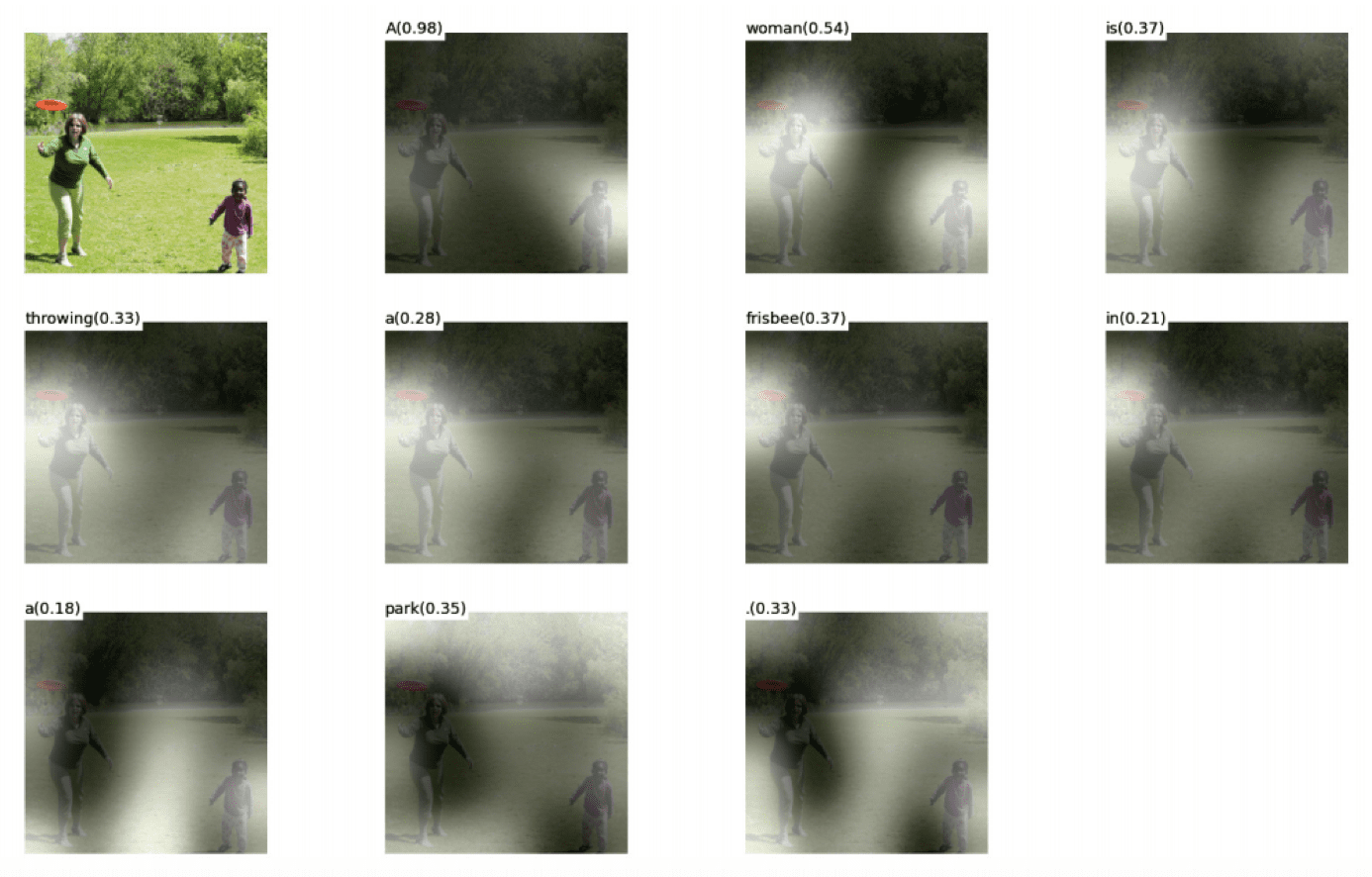Summary

• Signiﬁcant performance improvements
• Better performance over vector-based encodings
• Better performance with smaller training data sets
• Model interpretability